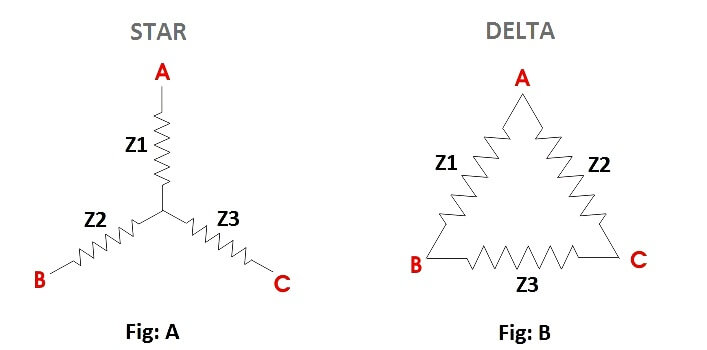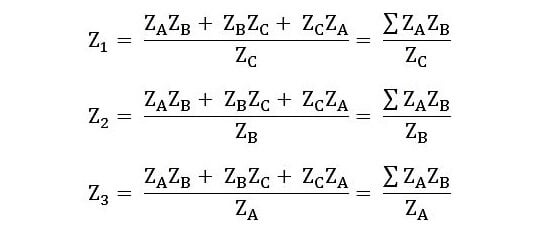# Star to Delta and Delta to Star Conversion Formula

The Conversion or transformation or replacement of the Star connected load network to a Delta connected network and similarly a Delta connected network to a Star Network is done by Star to Delta or Delta to Star Conversion.

#### Star to Delta ConversionIn star to delta conversion, the star connected load is to be converted into delta connection. Suppose we have a Star connected load as shown in the figure An above, and it has to be converted into a Delta connection as shown in figure B. The following Delta values are as follows.Hence, if the values of ZA, ZB and ZC are known, therefore by knowing these values and by putting them in the above equations, you can convert a star connection into a delta connection.

#### Delta to Star Conversion

Similarly, a Delta connection network is given as shown above, in figure B and it has to be transformed into a Star connection, as shown above, in the figure A. The following formulas given below are used for the conversion.If the values of Z1, Z2 and Z3 are given, then by putting these values of the Impedances in the above equations, the conversion of delta connection into star connection can be performed.

As Impedance (Z) is the vector quantity, therefore all the calculations are done in Polar and Rectangular form.